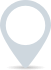# Rate of return

The rate of return (RoR) is a financial metric used to evaluate the profitability or performance of an investment over a specific period of time. It is expressed as a percentage and provides insight into the gain or loss generated from an investment relative to the initial amount invested.

There are several types of rates of return, each serving different purposes:

1. Simple rate of return: The simple rate of return is the most basic form of measuring investment performance. It is calculated using the following formula:Simple RoR = (net profit / initial investment) x 100%
1. Annualised rate of return: The annualised rate of return accounts for the time value of money and is useful for comparing investments with different time horizons
2. Total rate of return: The total rate of return encompasses all forms of return from an investment, including capital appreciation, dividends, interest, and other income. It is expressed as a percentage and is calculated using the following formula:Total RoR = (total gain / initial investment) x 100%
1. Risk-adjusted rate of return: This metric factors in the level of risk associated with an investment. It’s important because higher returns often come with higher risk.

Understanding the rate of return is crucial for investors, as it allows them to assess the performance of their investments, compare different investment opportunities, and make informed decisions about where to allocate their capital.### Clever finance tips and the latest news

#### delivered to your inbox, every week